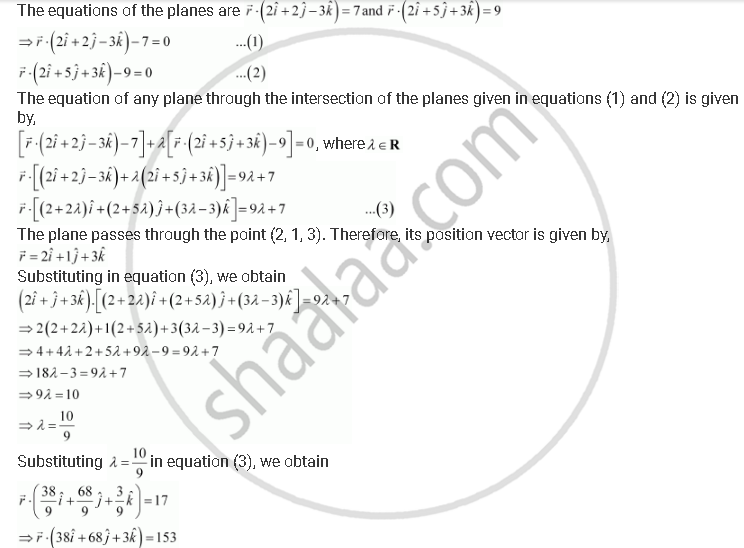Share

# Find the Vector Equation of the Plane Passing Through the Intersection of the Planes Vecr.(2hati + 2hatj - 3hatk) = 7, Vecr.(2hati + 5hatj + 3hatk) = 9 and Through the Point (2, 1, 3) - CBSE (Commerce) Class 12 - Mathematics

ConceptPlane Plane Passing Through the Intersection of Two Given Planes

#### Question

Find the vector equation of the plane passing through the intersection of the planes vecr.(2hati + 2hatj - 3hatk) = 7, vecr.(2hati + 5hatj + 3hatk) = 9 and through the point (2, 1, 3)

#### SolutionThis is the vector equation of the required plane.

Is there an error in this question or solution?

#### APPEARS IN

NCERT Solution for Mathematics Textbook for Class 12 (2018 to Current)
Chapter 11: Three Dimensional Geometry
Q: 10 | Page no. 493
Solution Find the Vector Equation of the Plane Passing Through the Intersection of the Planes Vecr.(2hati + 2hatj - 3hatk) = 7, Vecr.(2hati + 5hatj + 3hatk) = 9 and Through the Point (2, 1, 3) Concept: Plane - Plane Passing Through the Intersection of Two Given Planes.
S